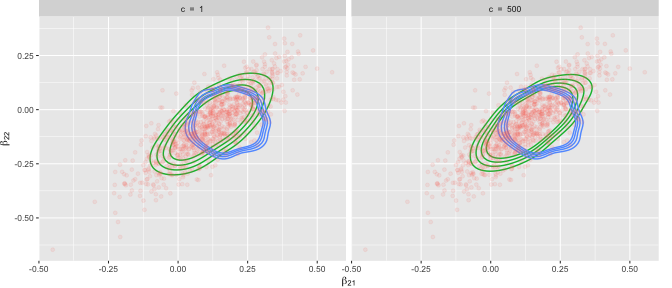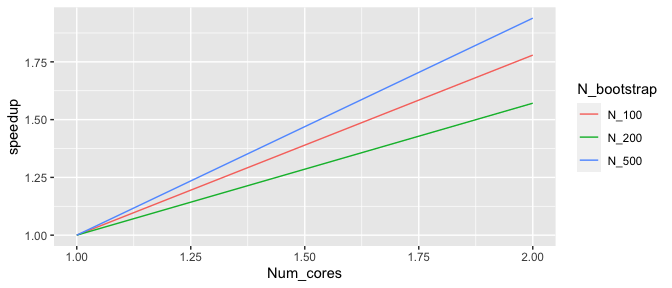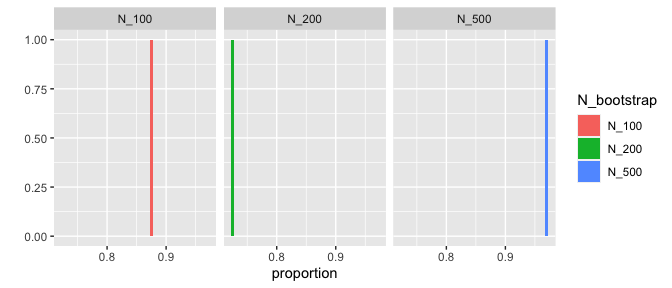# Introduction

This vignette shows the code generating figure 2 in Lyddon, Walker & Holmes, 2018, and also reuses material from that paper.

Bayesian learning is built on an assumption that the model space contains a true reflection of the data generating mechanism. This assumption is problematic, particularly in complex data environments. Here we present a Bayesian nonparametric approach to learning that makes use of statistical models, but does not assume that the model is true. Our approach admits a Monte Carlo sampling scheme that can afford massive scalability on modern computer architectures. The model-based aspect of learning is particularly attractive for regularizing nonparametric inference when the sample size is small, and also for correcting approximate approaches such as variational Bayes.

Here, we demonstrate the approach on a variational Bayes classifier.

We demonstrate this in practice through a variational Bayes logistic regression model fit to the Statlog German Credit dataset, containing 1000 observations and 25 covariates (including intercept), from the UCI ML repository and which ships with the package. The outcome is whether an individual has good credit rating.

# Setup

## Dependencies

We require our package and the rstan package for variational Bayes. Note that, for technical reasons (see StackOverflow issue), the rstan package needs to be loaded and attached, so we use library("rstan").

requireNamespace("PosteriorBootstrap", quietly = TRUE)

requireNamespace("dplyr", quietly = TRUE)
requireNamespace("ggplot2", quietly = TRUE)
requireNamespace("tibble", quietly = TRUE)

library("rstan")
#> rstan (Version 2.21.8, GitRev: 2e1f913d3ca3)
#> For execution on a local, multicore CPU with excess RAM we recommend calling
#> options(mc.cores = parallel::detectCores()).
#> To avoid recompilation of unchanged Stan programs, we recommend calling
#> rstan_options(auto_write = TRUE)

## Plotting functions

We define a ggproto object to compute the density of samples, and wrap it in a ggplot2 object:

#The first argument is required, either NULL or an arbitrary string.
stat_density_2d1_proto <- ggplot2::ggproto(NULL,
ggplot2::Stat,
required_aes = c("x", "y"),

compute_group = function(data, scales, bins, n) {
# Choose the bandwidth of Gaussian kernel estimators and increase it for
# smoother densities in small sample sizes
h <- c(MASS::bandwidth.nrd(data$x) * 1.5, MASS::bandwidth.nrd(data$y) * 1.5)

# Estimate two-dimensional density
dens <- MASS::kde2d(
data$x, data$y, h = h, n = n,
lims = c(scales$x$dimension(), scales$y$dimension())
)

# Store in data frame
df <- data.frame(expand.grid(x = dens$x, y = dens$y), z = as.vector(dens$z)) # Add a label of this density for ggplot2 df$group <- data$group # plot ggplot2::StatContour$compute_panel(df, scales, bins)
}
)

# Wrap that ggproto in a ggplot2 object
stat_density_2d1 <- function(data = NULL,
geom = "density_2d",
position = "identity",
n = 100,
...) {
ggplot2::layer(
data = data,
stat = stat_density_2d1_proto,
geom = geom,
position = position,
params = list(
n = n,
...
)
)
}

We define a shorthand function that appends all samples to a dataframe and is ready for plotting.

append_to_plot <- function(plot_df, sample, method,
concentration, x_index, y_index) {
new_plot_df <- rbind(plot_df, tibble::tibble(x = sample[, x_index],
y = sample[, y_index],
Method = method,
concentration = concentration))
return(new_plot_df)
}

## Sampling

We assign an independent normal prior with standard deviation 10 to each covariate, and generate 1000 posterior samples for each method: Bayesian logistic regression, variational Bayes, and Adaptive Non-Parametric Learning.

Note: variational Bayes in RStan is still experimental. We have had situations in the past where the same code with the same versions produces very different results. This thread on StackOverflow mentions this problem. In this code, variational Bayes provides the samples for our method, so the results may vary in the future because of the results from variational Bayes in RStan. Whether variational Bayes gives the expected answers or not, our approach will always give an answer that is in-between the results from variational Bayes and Bayesian logistic regression.

We tune the settings for the sampling with the number of draws, setting the seed, and getting the data:

seed <- 123
prior_sd <- 10
n_bootstrap <- 1000
german <- PosteriorBootstrap::get_german_credit_dataset()

For Bayesian logistic regression, we draw samples using the rstan package and the Bayesian logistic regression model that ships with this package:

if ("Windows" != Sys.info()["sysname"]) {
train_dat <- list(n = length(german$y), p = ncol(german$x), x = german$x, y = german$y, beta_sd = prior_sd)
stan_file <- PosteriorBootstrap::get_stan_file()
bayes_log_reg <- rstan::stan(stan_file, data = train_dat, seed = seed,
iter = n_bootstrap * 2, chains = 1)
stan_bayes_sample <- rstan::extract(bayes_log_reg)$beta } #> #> SAMPLING FOR MODEL 'bayes_logit' NOW (CHAIN 1). #> Chain 1: #> Chain 1: Gradient evaluation took 0.000198 seconds #> Chain 1: 1000 transitions using 10 leapfrog steps per transition would take 1.98 seconds. #> Chain 1: Adjust your expectations accordingly! #> Chain 1: #> Chain 1: #> Chain 1: Iteration: 1 / 2000 [ 0%] (Warmup) #> Chain 1: Iteration: 200 / 2000 [ 10%] (Warmup) #> Chain 1: Iteration: 400 / 2000 [ 20%] (Warmup) #> Chain 1: Iteration: 600 / 2000 [ 30%] (Warmup) #> Chain 1: Iteration: 800 / 2000 [ 40%] (Warmup) #> Chain 1: Iteration: 1000 / 2000 [ 50%] (Warmup) #> Chain 1: Iteration: 1001 / 2000 [ 50%] (Sampling) #> Chain 1: Iteration: 1200 / 2000 [ 60%] (Sampling) #> Chain 1: Iteration: 1400 / 2000 [ 70%] (Sampling) #> Chain 1: Iteration: 1600 / 2000 [ 80%] (Sampling) #> Chain 1: Iteration: 1800 / 2000 [ 90%] (Sampling) #> Chain 1: Iteration: 2000 / 2000 [100%] (Sampling) #> Chain 1: #> Chain 1: Elapsed Time: 1.17893 seconds (Warm-up) #> Chain 1: 1.3607 seconds (Sampling) #> Chain 1: 2.53963 seconds (Total) #> Chain 1: For variational Bayes, we obtain a mean-field variational Bayes sample using automatic differentiation variational inference using the rstan package and the same logistic regression model as above. The number of samples is n_bootstrap, as these samples serve for the Adaptive Non-Parametric Learning algorithm: if ("Windows" != Sys.info()["sysname"]) { stan_model <- rstan::stan_model(file = stan_file) stan_vb <- rstan::vb(object = stan_model, data = train_dat, seed = seed, output_samples = n_bootstrap) stan_vb_sample <- rstan::extract(stan_vb)$beta
}
#> Chain 1: ------------------------------------------------------------
#> Chain 1: EXPERIMENTAL ALGORITHM:
#> Chain 1:   This procedure has not been thoroughly tested and may be unstable
#> Chain 1:   or buggy. The interface is subject to change.
#> Chain 1: ------------------------------------------------------------
#> Chain 1:
#> Chain 1:
#> Chain 1:
#> Chain 1: Gradient evaluation took 0.00012 seconds
#> Chain 1: 1000 transitions using 10 leapfrog steps per transition would take 1.2 seconds.
#> Chain 1:
#> Chain 1:
#> Chain 1: Begin eta adaptation.
#> Chain 1: Iteration:   1 / 250 [  0%]  (Adaptation)
#> Chain 1: Iteration:  50 / 250 [ 20%]  (Adaptation)
#> Chain 1: Iteration: 100 / 250 [ 40%]  (Adaptation)
#> Chain 1: Iteration: 150 / 250 [ 60%]  (Adaptation)
#> Chain 1: Iteration: 200 / 250 [ 80%]  (Adaptation)
#> Chain 1: Success! Found best value [eta = 1] earlier than expected.
#> Chain 1:
#> Chain 1: Begin stochastic gradient ascent.
#> Chain 1:   iter             ELBO   delta_ELBO_mean   delta_ELBO_med   notes
#> Chain 1:    100         -593.338             1.000            1.000
#> Chain 1:    200         -591.578             0.501            1.000
#> Chain 1:    300         -593.057             0.335            0.003   MEDIAN ELBO CONVERGED
#> Chain 1:
#> Chain 1: Drawing a sample of size 1000 from the approximate posterior...
#> Chain 1: COMPLETED.
#> Warning: Pareto k diagnostic value is 1.23. Resampling is disabled. Decreasing
#> tol_rel_obj may help if variational algorithm has terminated prematurely.
#> Otherwise consider using sampling instead.

We now run the Adaptive Non-Parametric Learning algorithm, using the samples from variational Bayes, for two different values of the concentration hyper-parameter. Because our sampling method with c = 1000 takes longer than the timeout allowed on CRAN, we use only c = 500 for this vignette, but please see the GitHub page for the same figure as in the paper (with c = 1, c = 1,000, and c = 20,000).

if ("Windows" != Sys.info()["sysname"]) {
if (identical(Sys.getenv("NOT_CRAN", unset = "true"), "true")) {
concentrations <- c(1, 500)
anpl_samples <- list()
for (concentration in concentrations) {
anpl_sample <- PosteriorBootstrap::draw_logit_samples(x = german$x, y = german$y,
concentration = concentration,
n_bootstrap = n_bootstrap,
posterior_sample = stan_vb_sample,
threshold = 1e-8,
show_progress = TRUE)
anpl_samples[[toString(concentration)]] <- anpl_sample
}
}
}
#> ================================================================================================================================================================

## Preparing the plot

We now prepare a dataframe with all the samples ready for plotting.

if ("Windows" != Sys.info()["sysname"]) {
if (identical(Sys.getenv("NOT_CRAN", unset = "true"), "true")) {
# Initialise
plot_df <- tibble::tibble()

# Index of coefficients in the plot
x_index <- 21
y_index <- 22

# Create a plot data frame with all the samples
for (concentration in concentrations) {
plot_df  <- append_to_plot(plot_df, sample = anpl_samples[[toString(concentration)]],
method = "PosteriorBootstrap-ANPL",
concentration = concentration,
x_index = x_index, y_index = y_index)
plot_df  <- append_to_plot(plot_df, sample = stan_bayes_sample,
method = "Bayes-Stan",
concentration = concentration,
x_index = x_index, y_index = y_index)
plot_df  <- append_to_plot(plot_df, sample = stan_vb_sample,
method = "VB-Stan",
concentration = concentration,
x_index = x_index, y_index = y_index)
}
}
}

## Plotting the results

And now we plot the result of the algorithm:

if ("Windows" != Sys.info()["sysname"]) {
if (identical(Sys.getenv("NOT_CRAN", unset = "true"), "true")) {
ggplot2::ggplot(ggplot2::aes_string(x = "x", y = "y", colour = "Method"),
data = dplyr::filter(plot_df, plot_df$Method != "Bayes-Stan")) + stat_density_2d1(bins = 5) + ggplot2::geom_point(alpha = 0.1, size = 1, data = dplyr::filter(plot_df, plot_df$Method == "Bayes-Stan")) +
ggplot2::facet_wrap(~concentration, nrow = 1,
scales = "fixed",
labeller = ggplot2::label_bquote(c ~" = "~
.(concentration))
) +
ggplot2::theme_grey(base_size = 8) +
ggplot2::xlab(bquote(beta[.(x_index)])) +
ggplot2::ylab(bquote(beta[.(y_index)])) +
ggplot2::theme(legend.position = "none",
plot.margin = ggplot2::margin(0, 10, 0, 0, "pt"))
}
}
#> Warning: aes_string() was deprecated in ggplot2 3.0.0.
#> ℹ Please use tidy evaluation ideoms with aes()The Non-parametric update effectively corrects the variational Bayes approximation for small values of the concentration c. As we increase the concentration parameter, the samples drawn with our method move closer to those from the variational Bayes approximation, showing that the concentration parameter is an effective sample size tuning of the weight to give to the data compared to the approximate model.

Of course, local variational methods can provide more accurate posterior approximations to Bayesian logistic posteriors, though these too are approximations, that our algorithm can correct.

# Parallelisation and performance

Our construction admits a trivially parallelisable sampler once the parametric posterior samples have been generated (or if the concentration c equals 0). Note that, although some algorithms to generate the parametric posterior samples may be parallelisable, above we use a Markov Chain-Monte Carlo algorithm from RStan, which is not parallelisable. We emphasise that the part of our algorithm that benefits from modern parallel computer architectures happens after we have the posterior samples (or if c=0).

We now illustrate the parallelisation speedup. The calculation of the expected speedup depends on the number of bootstrap samples and the number of processors. It also depends on the system: it is larger on macOS than on Linux, with some variation depending on the version of R.

Due to limitations in the R workflow, this vignette is limited to one or two cores, but see the GitHub page for a plot up to 64 cores.

### Ahmdal’s law

Fixing the number of samples corresponds to Ahmdal’s law, or the speedup in the task as a function of the number of processors. The speedup S_latency of N processors is defined as the duration of the task with one core divided by the duration of the task with N processors. We compute the duration for one or two cores and for several values of bootstrap samples.

if ("Windows" != Sys.info()["sysname"] ) {
speedups  <- data.frame(stringsAsFactors = FALSE)
max_cores <- 2
n_bootstrap_array <- c(1e2, 2e2, 5e2)

for (n_bootstrap in n_bootstrap_array) {
one_core_duration <- NULL
for (num_cores in seq(1, max_cores)) {

start <- Sys.time()
anpl_samples <- PosteriorBootstrap::draw_logit_samples(x = german$x, y = german$y,
concentration = 1,
n_bootstrap = n_bootstrap,
gamma_mean = rep(0, ncol(german$x)), gamma_vcov = diag(1, ncol(german$x)),
threshold = 1e-8,
num_cores = num_cores)
lap <- as.double((Sys.time() - start), units = "secs")
if (1 == num_cores) {
one_core_duration <- lap
}
speedups <- rbind(speedups, c(num_cores, n_bootstrap, one_core_duration / lap))
}
names(speedups) <- c("Num_cores", "N_bootstrap", "speedup")
}

# Convert n_bootstrap to strings for ggplot2 to arrange them into groups
speedups$N_bootstrap <- paste0("N_", speedups$N_bootstrap)

ggplot2::ggplot(data = speedups,
ggplot2::aes(x = Num_cores, y = speedup)) +
ggplot2::geom_line(ggplot2::aes(colour = N_bootstrap))
}We invert Ahmdal’s law to compute the proportion of the execution time that is parallelisable from the speedup as:

$p = \frac{\frac{1}{S_{latency}}} - 1}{\frac{1}{s} - 1}$

where $$S_{latency}$$ is the theoretical speedup of the whole task in Ahmdal’s law and the observed speedup here, and $$s$$ is the speedup of the part of the task that can be parallelised, and thus equal to the number of processors. Calculating this value for the durations from 1 to 8 cores, we obtain this plot, where the proportion of the code that can be parallelised is high:

if ("Windows" != Sys.info()["sysname"] ) {
library("gridExtra")

# Remove single core speedup, where the proportion is not defined
speedups <- speedups[1 != speedups$Num_cores, ] speedups$proportion <- (1 / speedups$speedup - 1) / (1 / speedups$Num_cores - 1)

ggplot2::qplot(proportion, data = speedups, fill = N_bootstrap, binwidth = 0.005) +
ggplot2::facet_wrap(facets = ~N_bootstrap)
}
#> Warning: qplot() was deprecated in ggplot2 3.4.0.